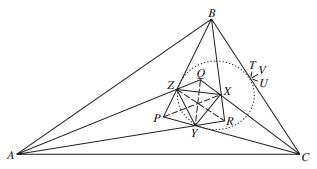# Morley's Theorem, a Proof

### Brian Stonebridge 2009

In the diagram below, the near trisectors of the internal angles at the vertices $A,$ $B,$ and $C$ of a triangle meet in $X,$ $Y,$ and $Z.$ Morley's theorem states that the triangle $XYZ$ is equilateral.Using the notation in the diagram, since, in $\Delta ABC,$3\alpha + 3\beta + 3\gamma = \pi ,\alpha + \beta + \gamma = \pi /3.$Start with an arbitrary equilateral triangle$XYZ.$. Let$P,Q,R$be point on the altitudes$\Delta XYZ$(produced) such that$\displaystyle\angle XPY=\angle XPZ=\alpha+\frac{\pi}{6},\\ \angle YQZ=\angle YQX=\beta+\frac{\pi}{6},\\ \angle ZRX=\angle ZRY=\gamma+\frac{\pi}{6}. $Define$A$to be the intersection of$QZ$and$RY,B$the intersection of$RX$and$PZ,$and$C$that of$PY$and$QX.$Then in quadrilateral$XRAQ,\displaystyle\angle AQX=2\beta +\frac{\pi}{3},\displaystyle\angle ARX=2\gamma +\frac{\pi}{3},$and$\displaystyle\angle QXR=2\alpha +\beta +\gamma+\frac{\pi}{3}.$It follows that$\angle ZAY=\alpha.$Similarly,$\angle XBZ =\beta$and$\angle YCX=\gamma.$Draw circle with center$X$touching$PB$and, since$PX$bisects$\angle BPC,$it also touches$PC.$Next, draw tangents$BT$and$CU$, set$V$as the intersection of the two lines. Then,$\angle XBT=\angle XBZ=\beta$and$\angle XCU =\angle XCY=\gamma.$Now, the sum of angles$P,B,$and$C$in quadrilateral$PBVC$equals$\displaystyle\angle QXR=2\alpha+\frac{\pi}{3} +2\beta +2\gamma=\pi,$implying that$\angle TVU=0.$In other words,$BTVUC$is a straight line so that$T$and$U$coincide in$V.$It follows that$\angle XBC=\beta$and$\angle XCB=\gamma.$In the same manner the angles of triangles$YCA$and$ZAB$are determined, letting one to conclude that triangle$ABC$has angles$3\alpha,3\beta,$and$3\gamma.\$ It may be scaled (if need be) to coincide with the original triangle.

1. B. Stonebridge, A Simple Geometric Proof of Morley's Trisector Theorem, Applied Probability Trust, 2009### Morley's Miracle

#### Invalid proofs